Geometric sums: solve4 variable algebraic equations in 2 minutes - Play this Free Online Education Game which is a 2 minute game, addition game, algebra game, education game, fast game, geometry game, hard game, kids game, math game, small game, sum game, time limit and all free games on Fupa Games

# "Geometric sums: solve4 variable algebraic equations in 2 minutes"A Free Online Game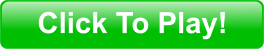Free Game Plays: 3203  Online Game Rating: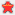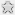Online Bookmark:## Geometric sums: solve4 variable algebraic equations in 2 minutes Online Information

Geometric sums: solve4 variable algebraic equations in 2 minutes Category: Online Education Games
Description: Geometric shapes represent variables, watch the patterns carefully and solve the equations, you will have a penalty for wrong answers, help us improve Geometric sums game with your input This game is online.
Tags: , , , , , , , , , , ,
Developer: fopgames
Instructions: Check inside the game for instructions on how to play Geometric sums: solve4 variable algebraic equations in 2 minutes.

 Link Code: | Embed Code: | IFrame Code:
Geometric sums: solve4 variable algebraic equations in 2 minutes

Click to play this game. Add this game to your web page! Copy this code to your page### Geometric sums: solve4 variable algebraic equations in 2 minutes Game Comments

Recent Online Scores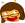adrifra-50fopgames4820Tashe-1080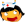TERRI169310TERRI168320TERRI164910TERRI164880TERRI16430

If you liked Geometric sums: solve4 variable algebraic equations in 2 minutes you might also like this game:DMCA | Privacy | Sitemap